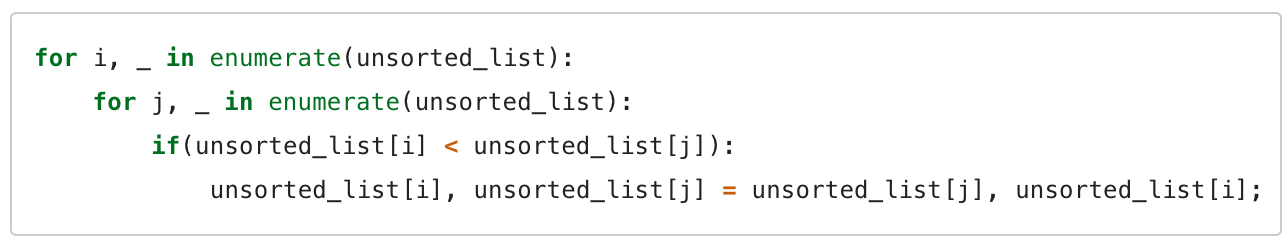# RandomlyUnique

## Reinforcement Sort

You know Quicksort and Merge sort and Timsort and Shell sort
Heapsort and Bubble sort and Insertion sort and Selection sort
But do you recall the most god-awful sort of all?

A Reinforcement Sort (a sort done through reinforcement(ish) learning) is the Nickelback of sorting algorithms - a perfect horrible and awesome superposition. This rockstar was developed using an epsilon greedy Q-learning approach with the aim of generating the best python sorting script. This mainly involved combining code fragments together and giving rewards based on how well these scripts sort a list. The exact setup is summarized as follows:

1) : A binary array chad-kroegered from a md5 hash of the python code string

``````import hashlib
import numpy as np

ARRAY_INPUT_SIZE = 128

def oh_god_why(code):
'''
This converts the code string (state) to a binary array (for the neural net)
'''

state_hash = hashlib.md5(code)
state_int = int(state_hash.hexdigest(), 16)
state_bin = bin(state_int)
state_str = str(state_bin)[2:].zfill(ARRAY_INPUT_SIZE)
state_array = np.array(list(state_str))

return state_array.reshape(1, ARRAY_INPUT_SIZE)
``````

2) : I figured sorting will require some looping, swapping and probably a conditional or two, so I made these actions on a state

``````from itertools import product

class Action(object):
def __init__(self, code):
self.code = code
self.start_list = 'unsorted_list'

def _lookup_element(self, var):
return "{list}[{var}]".format(**{
'list': self.start_list,
'var': var
})

def loop_through_elements(self, var, _):
self.code += "for {var}, _ in enumerate({list}):\n\t".format(**{
'list': self.start_list,
'var': var,
})

def swap(self, var1, var2):
self.code += "{ele1}, {ele2} = {ele2}, {ele1};".format(**{
'ele1': self._lookup_element(var1),
'ele2': self._lookup_element(var2)
})

def compare_elements(self, var1, var2):
self.code += "\tif( {ele1} < {ele2} ):".format(**{
'ele1': self._lookup_element(var1),
'ele2': self._lookup_element(var2)
})

def generate_actions():
'''
Gives all the unhidden methods (possible actions) from the Action class
'''
actions = Action.__dict__.keys()
actions = filter(lambda method: not method.startswith('_'), actions)
vars = ['i', 'j']
possible_actions = [e for e in product(actions, vars, vars) if e != e]

return possible_actions

def performAction(state, action):
'''
Takes the current state (the code string) and applies an action
'''
a = Action(state)
getattr(a, action)(*action[1:])
return a.code
``````

3) : The state receives a score of -1 if the python code doesn't run, -10 is the code is over 300 characters or +1000 if the list is correctly sorted.

``````import random

LIST_LENGTH = 1000
START_LIST = range(LIST_LENGTH)
random.shuffle(START_LIST)

TEMPLATE = '''
def sort(unsorted_list):
{0}
return unsorted_list
sorted_list = sort({1})
'''

def append_definition(code, start_list):
"""
This takes the above TEMPLATE string and wraps it around the code string
"""
return TEMPLATE.format(code, start_list)

def calc_reward(code):
"""
Rewards based on the current code state:
1) Code correctly sorts the list: 1000
2) Code runs: 0
3) Code doesn't run: -1
4) Code is over 300 characters: -10
"""
if len(code) > 300:
return -10

try:
exec append_definition(code, START_LIST)

score = 0
if sorted_list == range(LIST_LENGTH):
score = 1000

except:
score = -1

return score
``````

After up a neural net, I received this optimal sort:

``````import random
import numpy as np
from keras.models import Sequential
from keras.layers.core import Dense, Activation
from keras.optimizers import RMSprop
from action import performAction, oh_god_why, generate_actions, ARRAY_INPUT_SIZE
from reward import calc_reward

EPOCHS = 20000
GAMMA = 0.9

def setup_network(num_actions):
'''
This defines a neural net since that's the cool thing to do nowadays
'''
model = Sequential()

rms = RMSprop()
model.compile(loss='mse', optimizer=rms)

return model

def learn_best_sort(actions):
'''
Reinforcement learning via an epsilon greedy strategy
'''
model = setup_network(len(actions))
epsilon = 1

for epoch in range(EPOCHS):
if epoch % 250 == 0:
print 'Epoch = ', epoch

state = ''
while (True):
q = model.predict(oh_god_why(state))

if (random.random() < epsilon):
action = np.random.randint(0, len(actions))
else:
action = (np.argmax(q))

updated_state = performAction(state, actions[action])
reward = calc_reward(updated_state)

new_q = model.predict(oh_god_why(updated_state))
q_max = np.max(new_q)

if reward == -1:
update = reward + (GAMMA * q_max)
else:
update = reward

q[action] = update
model.fit(oh_god_why(state), q, verbose=0, nb_epoch=1)
state = updated_state

if reward != -1:
break

if epoch < EPOCHS - 1:
epsilon -= 1.0 / EPOCHS
else:
print state

if __name__ == '__main__':
actions = generate_actions()
learn_best_sort(actions)
``````Now you may be telling yourself that this looks exactly like a Bubble Sort, but I assure you it's not. A Bubble Sort has a reasonable time complexity of O(n^2), but a Reinforcement Sort runs in O(oh god, why am I doing this).